Home

# Control of viscous instability by variation of injection rate in a fluid with time-dependent rheology

## Abstract

Using variational calculus, we investigate the time-dependent injection rate that minimises the growth of the Saffman–Taylor instability when a finite volume of fluid is injected in a finite time,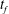$t_{f}$ , into a Hele-Shaw cell. We first consider a planar interface, and show that, with a constant viscosity ratio, the constant injection rate is optimal. When the viscosity of the displacing fluid,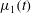$\unicode[STIX]{x1D707}_{1}(t)$ , gradually increases over time, as may occur with a slowly gelling polymer solution, the optimal injection rate,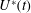$U^{\ast }(t)$ , involves a gradual increase in the flow rate with time. This leads to a smaller initial value of flow rate than the constant injection rate, finishing with a larger value. Such optimisation can lead to a substantial suppression of the instability as compared to the constant injection case if the characteristic gelling time is comparable to$t_{f}$ . In contrast, for either relatively slow or fast gelling, there is much less benefit in selecting the optimal injection rate,$U^{\ast }(t)$ , as compared to the constant injection rate. In the case of a constant injection rate from a point source,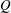$Q$ , then with a constant viscosity ratio the fastest-growing perturbation on the radially spreading front involves axisymmetric modes whose wavenumber increases with time. Approximating the discrete azimuthal modes by a continuous distribution, we find the injection rate that minimises growth,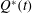$Q^{\ast }(t)$ . We find that there is a critical time for injection,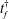$t_{f}^{\dagger }$ , such that if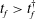$t_{f}>t_{f}^{\dagger }$ then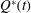$Q^{\ast }(t)$ can be chosen so that the interface is always stable. This critical time emerges from the case with an injection rate given by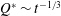$Q^{\ast }\sim t^{-1/3}$ . As the total injection time is reduced to values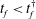$t_{f}<t_{f}^{\dagger }$ , the system becomes progressively more unstable, and the optimal injection rate for an idealised continuous distribution of azimuthal modes asymptotes to a flow rate that increases linearly with time. As for the one-dimensional case, if the viscosity of the injection fluid gradually increases over time, then the optimal injection rate has a smaller initial value but gradually increases to larger values than for the analogous constant viscosity problem. If the displacing fluid features shear-thinning rheology, then the optimal injection rate involves a smaller flow rate at early times, although not as large a reduction as in the Newtonian case, and a larger flow rate at late times, although not as large an increase as in the Newtonian case.

## References

Hide All
MathJax
MathJax is a JavaScript display engine for mathematics. For more information see http://www.mathjax.org.

# Control of viscous instability by variation of injection rate in a fluid with time-dependent rheology

## Metrics

### Full text viewsFull text views reflects the number of PDF downloads, PDFs sent to Google Drive, Dropbox and Kindle and HTML full text views.

Total number of HTML views: 0
Total number of PDF views: 0 *Loading metrics...

### Abstract viewsAbstract views reflect the number of visits to the article landing page.

Total abstract views: 0 *Loading metrics...

* Views captured on Cambridge Core between <date>. This data will be updated every 24 hours.

Usage data cannot currently be displayed.